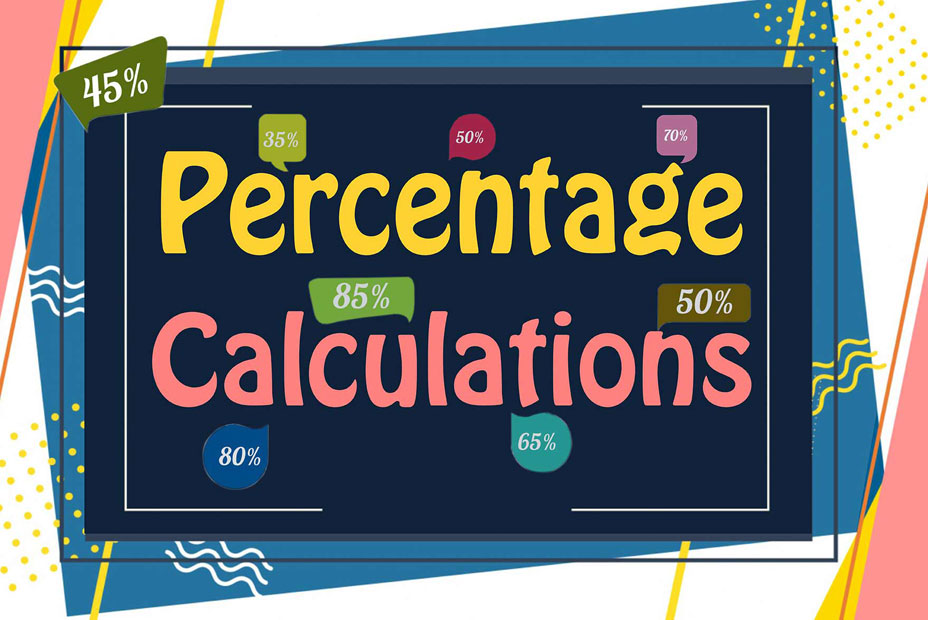## How to Calculate Percentage?

How many of you are aware that the word percent is derived from the Latin word "per centum"? It is also clear that if you break down the word "percent" into the two words "per" and "cent," the meaning becomes pretty clear: it means by a hundred percent. In this case, we can conclude that percentages are nothing more than fractions with denominators equal to $$100$$ in all instances.

### Calculation of Percentage

Now that we know what is percentage, let’s find out how to calculate it with ease. In percentage problems, we basically have to find the part/share of a whole in terms of $$100$$. This we can do in the following 2 ways:

• Firstly, we can apply the unitary method.
• Secondly, we take the fraction in consideration and change its denominator to $$100$$.

A thing to note is that the second method can only be effectively used when the denominator of the fraction is a factor of $$100$$. If this isn’t the case, we will always resort to the first method.

Example 1: $$6$$ is what percentage of $$24$$?
So, for this question, we first make the fraction as $$\frac{6}{24}$$ , and then since the fraction can be simplified, we simplify it to $$\frac{1}{4}$$ .
Next, we will multiply it by $$100$$ to get the percentage as $$\frac{1}{4} \times 100 \ = \ 25%$$

Example 2: $$9$$ is what percentage of $$63$$?
So, for this question, we first make the fraction as $$\frac{9}{63}$$ , and then since the fraction can be simplified, we simplify it to $$\frac{1}{7}$$ .
Next, we will multiply it by $$100$$ to get the percentage as $$\frac{1}{7} \times 100 \ = \ 14.28%$$

Example 3: $$27$$ is what percentage of $$81$$?
So, for this question, we first make the fraction as $$\frac{27}{81}$$ , and then since the fraction can be simplified, we simplify it to $$\frac{1}{3}$$ .
Next, we will multiply it by $$100$$ to get the percentage as $$\frac{1}{3} \times 100 \ = \ 33.33%$$ *
* Also, remember that the result of such calculations can depend on the degree of precision or the number of decimal places you are rounding to. In many cases, $$33.33$$ might be rounded to $$33.3$$  or even $$33$$ depending on the context.

### Exercises for Percentage Calculations

1) $$4.2$$ is what percentage of $$12?$$$$\ \Rightarrow \$$

2) $$9.45$$ is what percentage of $$27?$$$$\ \Rightarrow \$$

3) $$0.95$$ is what percentage of $$19?$$$$\ \Rightarrow \$$

4) $$24.6$$ is what percentage of $$41?$$$$\ \Rightarrow \$$

5) $$15.3$$ is what percentage of $$34?$$$$\ \Rightarrow \$$

6) $$16.8$$ is what percentage of $$56?$$$$\ \Rightarrow \$$

7) $$53.55$$ is what percentage of $$63?$$$$\ \Rightarrow \$$

8) $$74.1$$ is what percentage of $$78?$$$$\ \Rightarrow \$$

9) $$63.75$$ is what percentage of $$85?$$$$\ \Rightarrow \$$

10) $$80$$ is what percentage of $$100?$$$$\ \Rightarrow \$$

1) $$4.2$$ is what percentage of $$12?$$$$\ \Rightarrow \ \color{red}{\frac{4.2 \times 100}{12} \ = \ 35}$$
2) $$9.45$$ is what percentage of $$27?$$$$\ \Rightarrow \ \color{red}{\frac{9.45 \times 100}{27} \ = \ 35}$$
3) $$0.95$$ is what percentage of $$19?$$$$\ \Rightarrow \ \color{red}{\frac{0.95 \times 100}{19} \ = \ 5}$$
4) $$24.6$$ is what percentage of $$41?$$$$\ \Rightarrow \ \color{red}{\frac{24.6 \times 100}{41} \ = \ 60}$$
5) $$15.3$$ is what percentage of $$34?$$$$\ \Rightarrow \ \color{red}{\frac{15.3 \times 100}{34} \ = \ 45}$$
6) $$16.8$$ is what percentage of $$56?$$$$\ \Rightarrow \ \color{red}{\frac{16.8 \times 100}{56} \ = \ 30}$$
7) $$53.55$$ is what percentage of $$63?$$$$\ \Rightarrow \ \color{red}{\frac{53.55 \times 100}{63} \ = \ 85}$$
8) $$74.1$$ is what percentage of $$78?$$$$\ \Rightarrow \ \color{red}{\frac{74.1 \times 100}{78} \ = \ 95}$$
9) $$63.75$$ is what percentage of $$85?$$$$\ \Rightarrow \ \color{red}{\frac{63.75 \times 100}{85} \ = \ 75}$$
10) $$80$$ is what percentage of $$100?$$$$\ \Rightarrow \ \color{red}{\frac{80 \times 100}{100} \ = \ 80}$$

## Percentage Calculations Practice Quiz

### STAAR Grade 8 Math Study Guide

$19.99$14.99

### PSAT Math Practice Workbook

$25.99$14.99

### HSPT Math for Beginners

$24.99$14.99

### SSAT Middle Level Math Comprehensive Prep Bundle

$89.99$49.99